# NCERT Solutions For Class 10 Maths Chapter 2 Exercise 2.3 – Polynomials

Download NCERT Solutions For Class 10 Maths Chapter 2 Exercise 2.3 – Polynomials. This Exercise contains 5 questions, for which detailed answers have been provided in this note. In case you are looking at studying the remaining Exercise for Class 10 for Maths NCERT solutions for other Chapters, you can click the link at the end of this Note.### NCERT Solutions For Class 10 Maths Chapter 2 Exercise 2.3 – Polynomials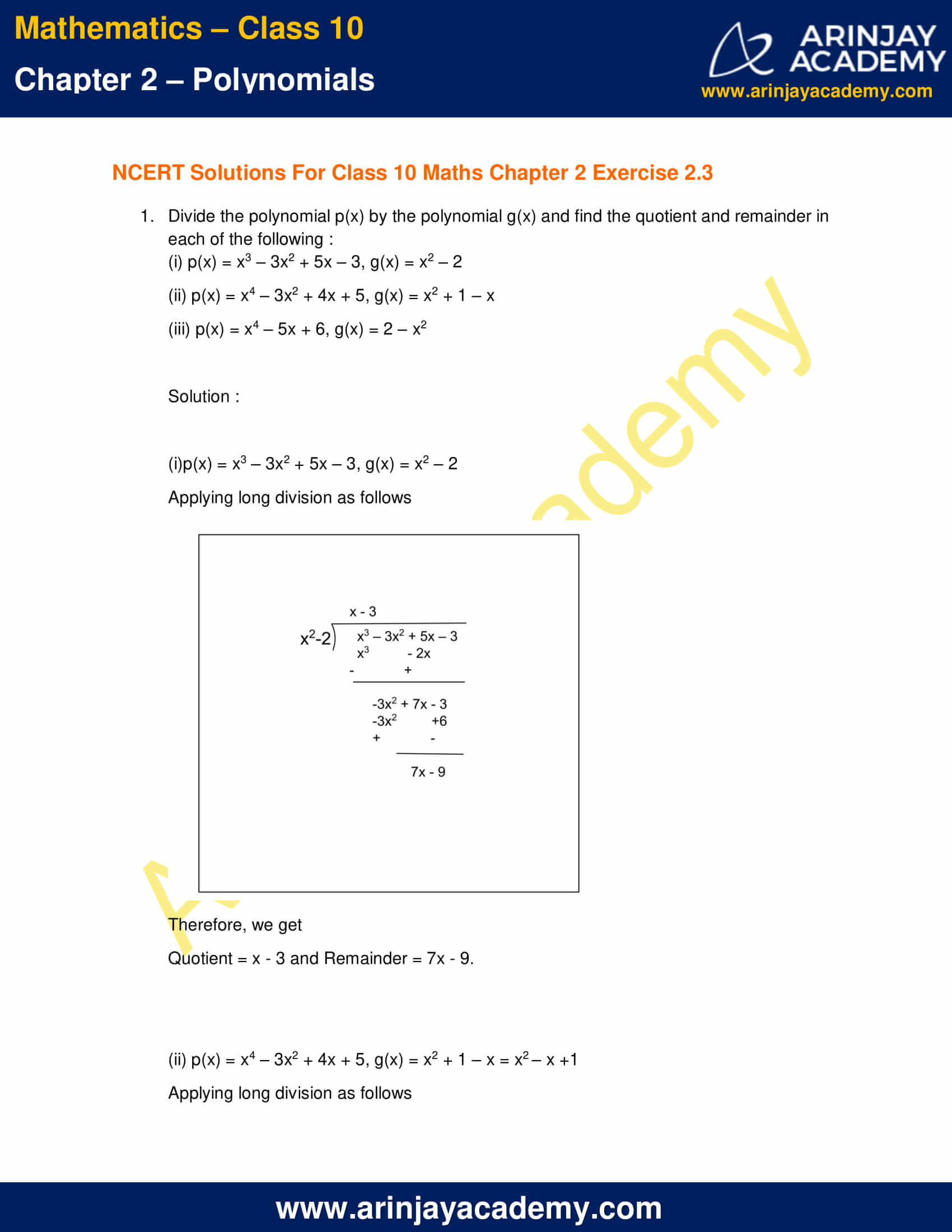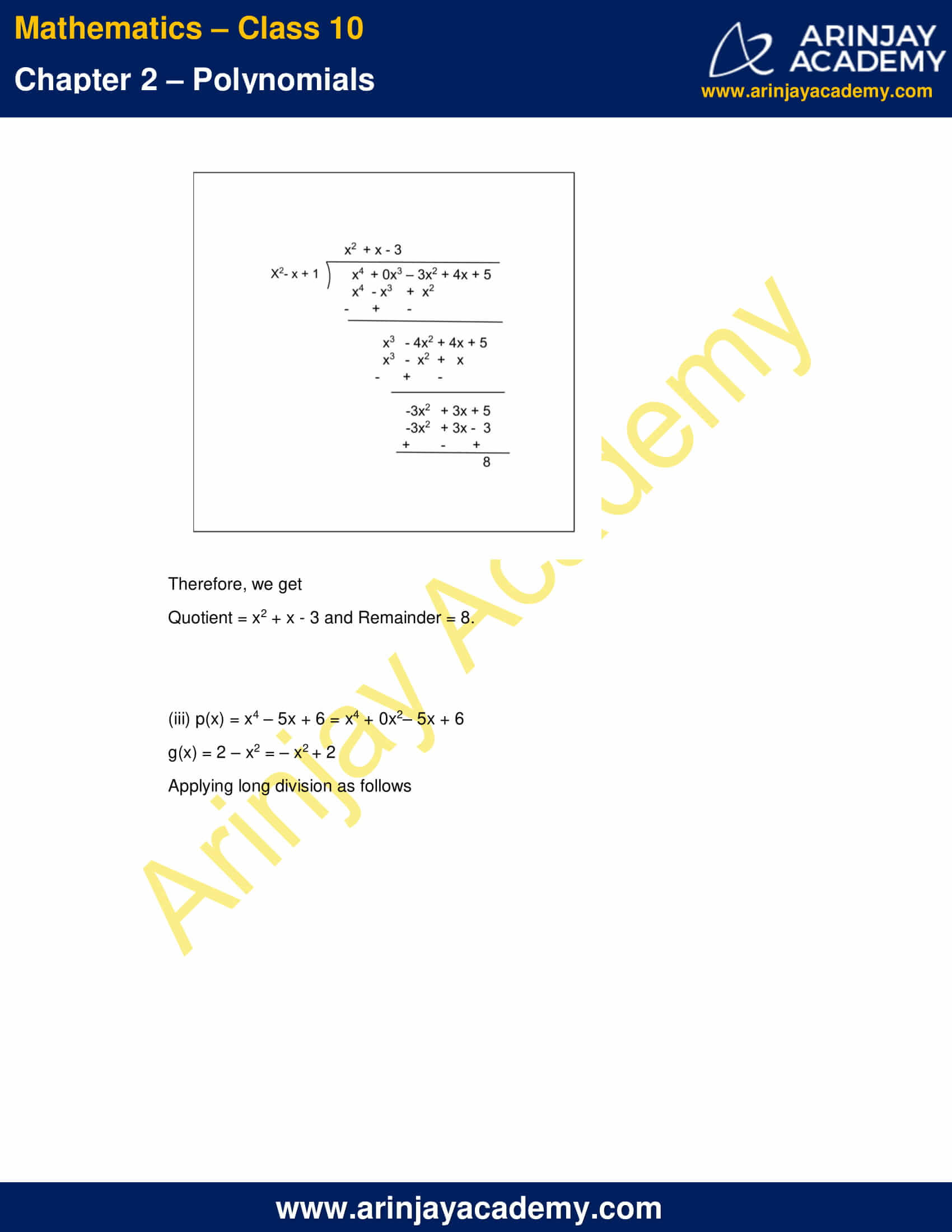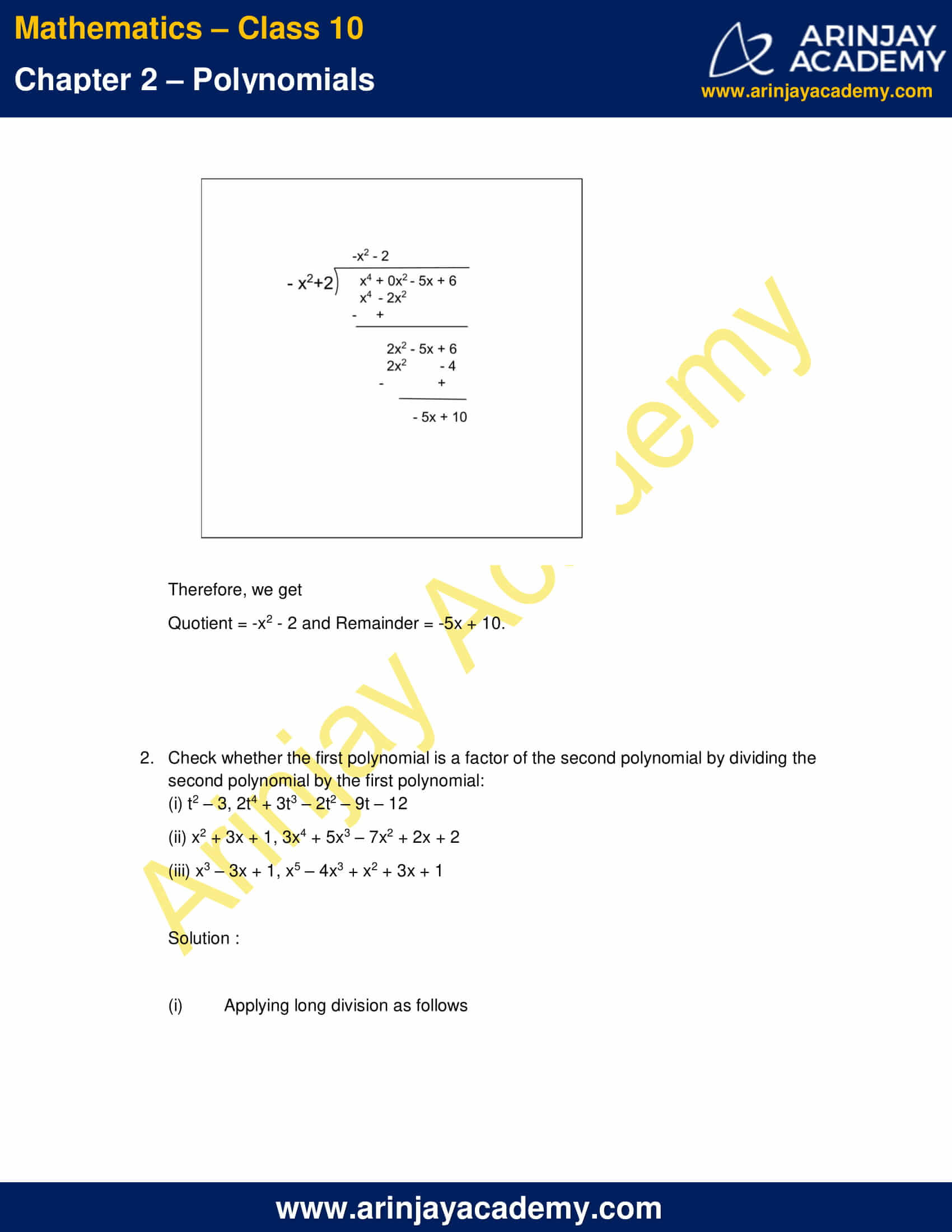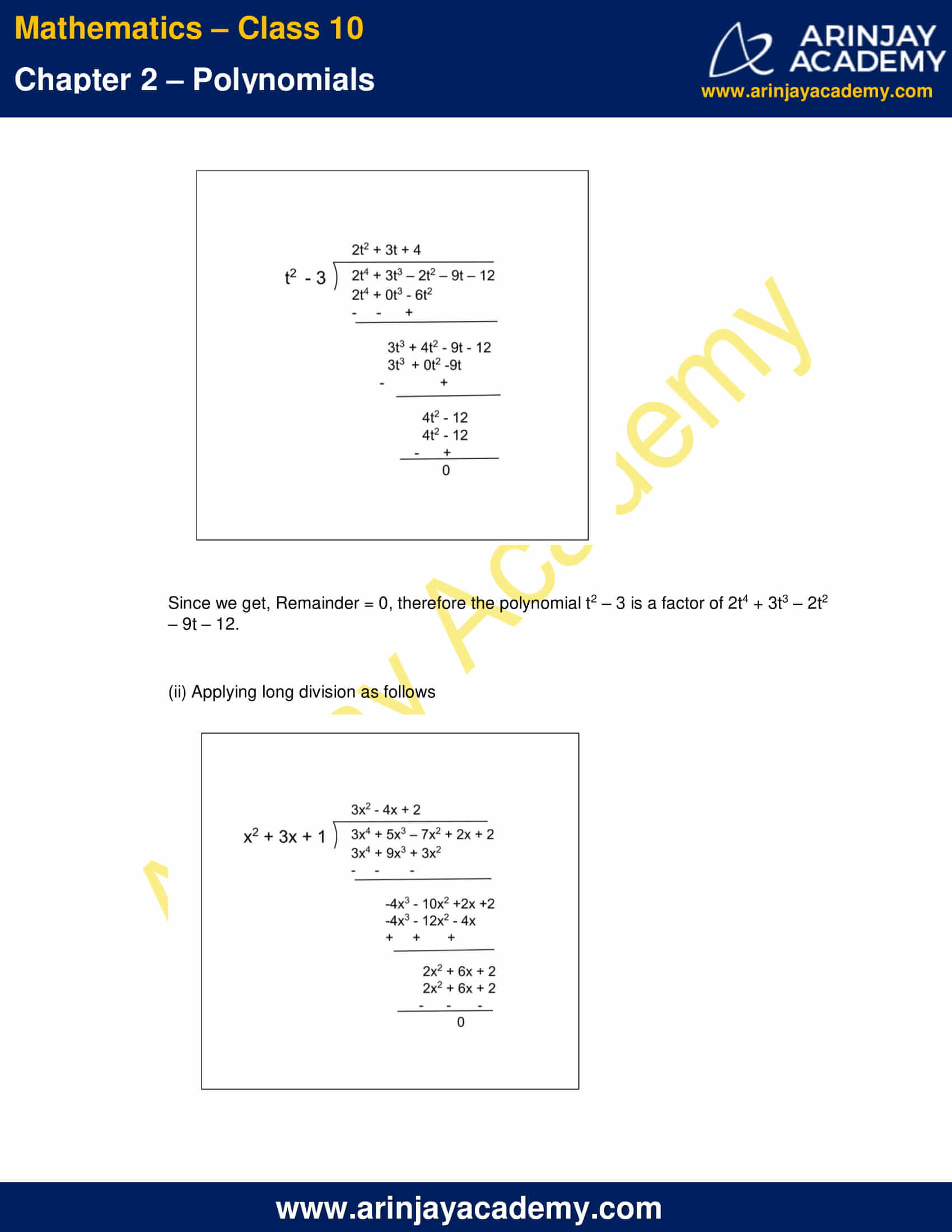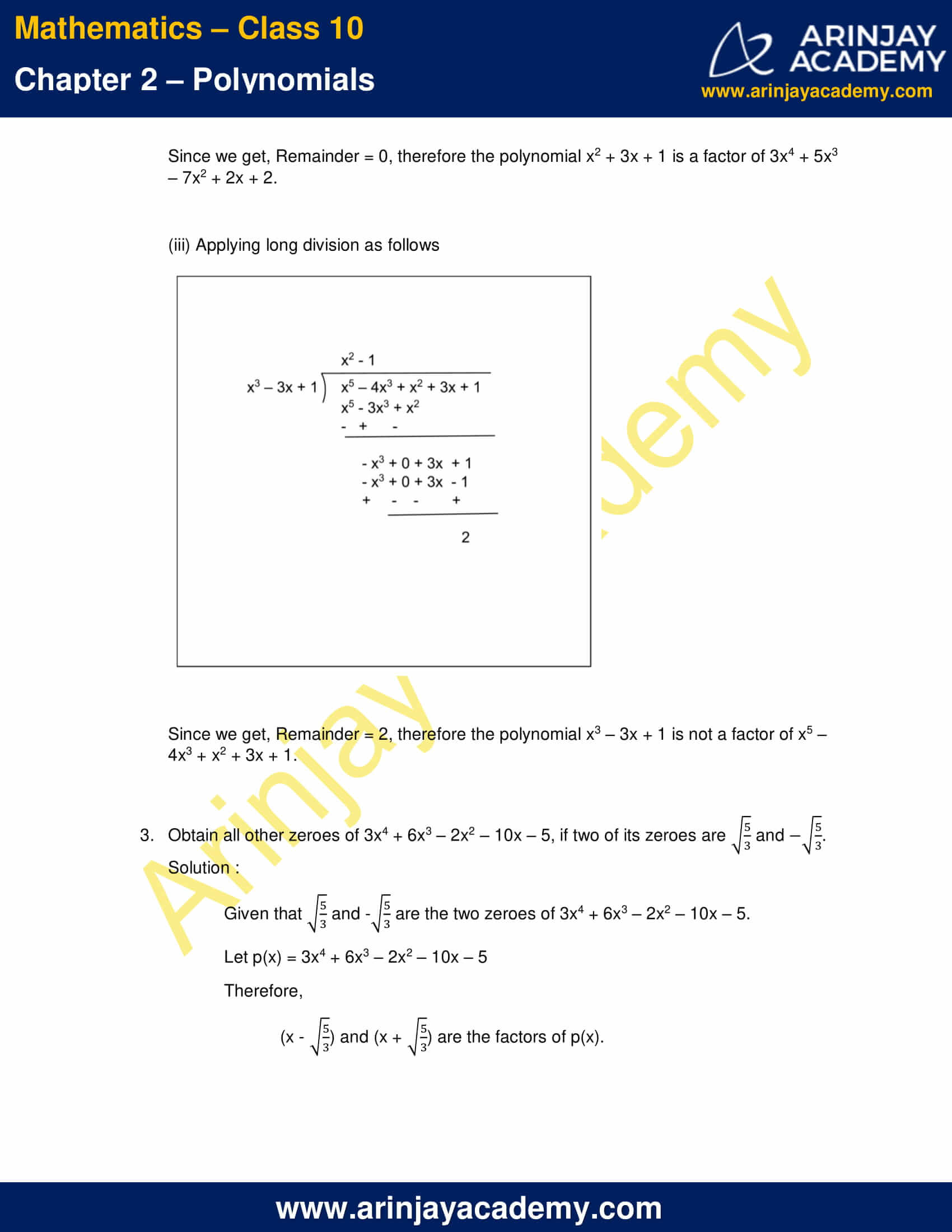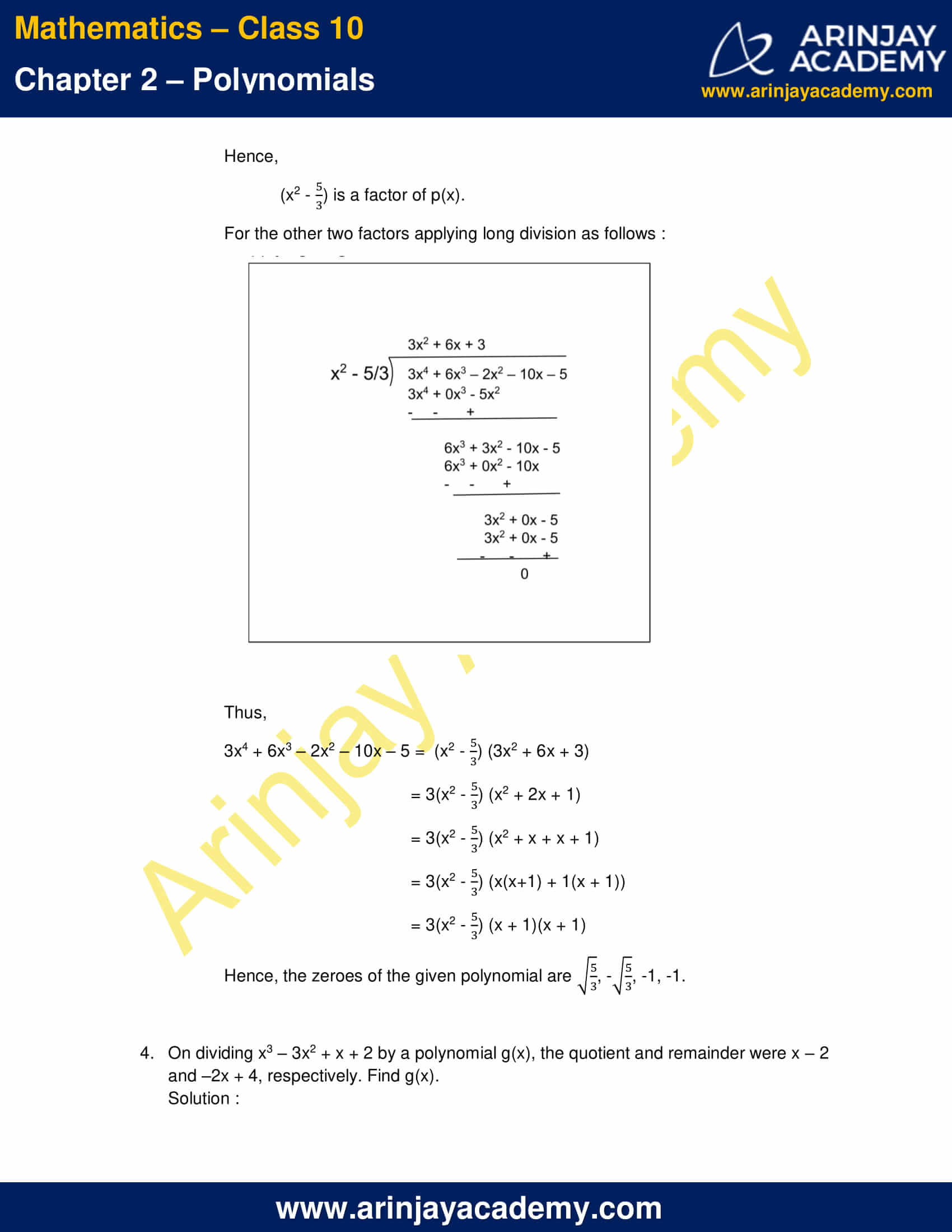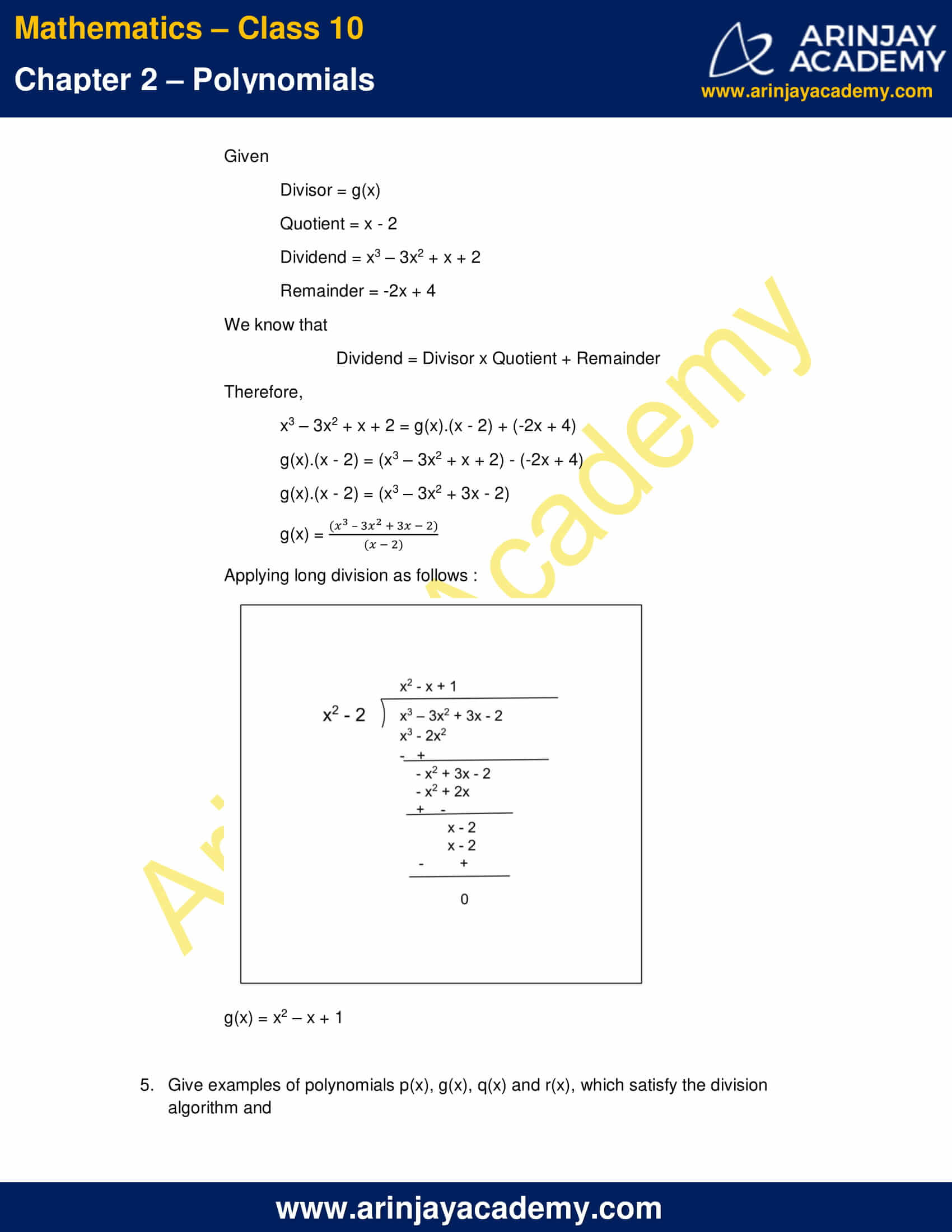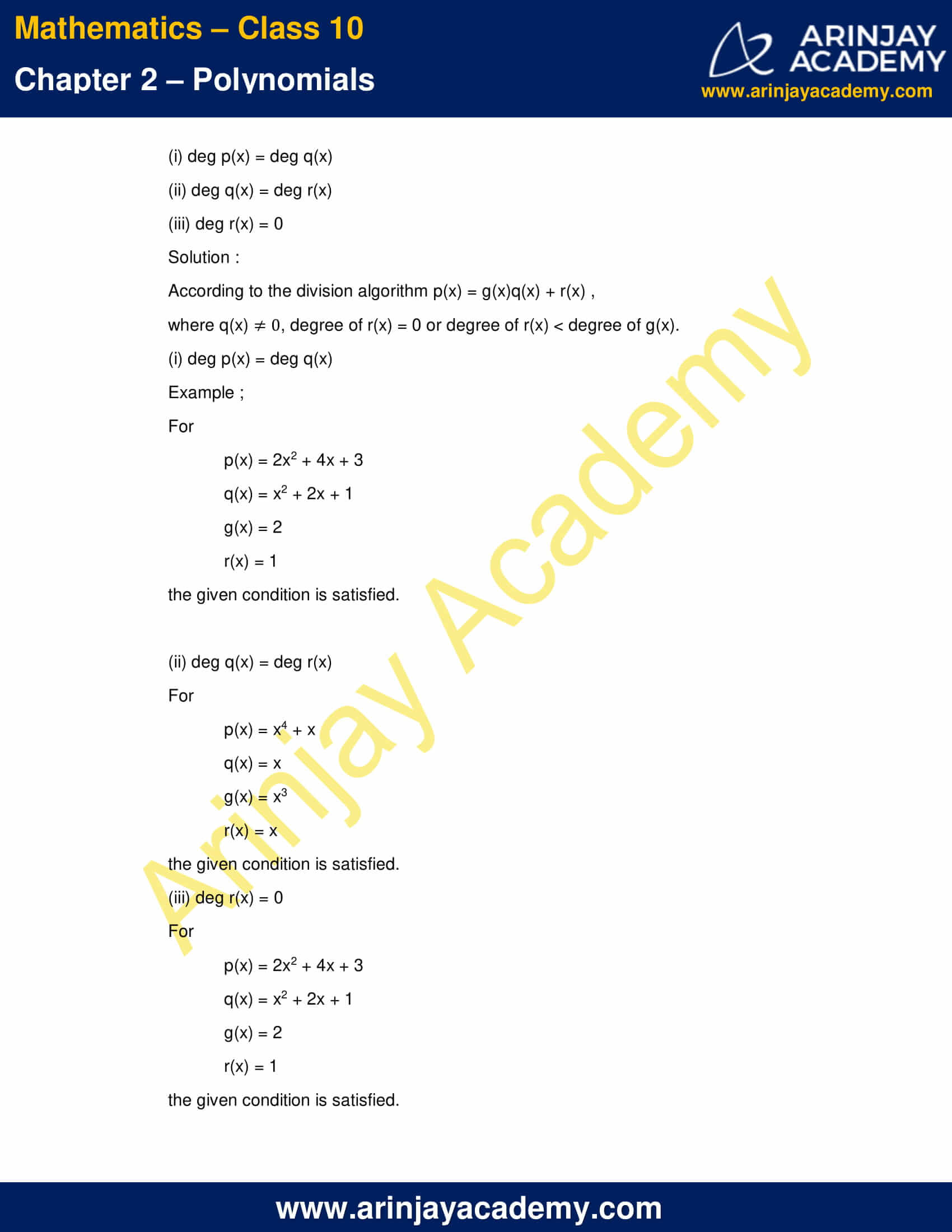NCERT Solutions For Class 10 Maths Chapter 2 Exercise 2.3 – Polynomials

1. Divide the polynomial p(x) by the polynomial g(x) and find the quotient and remainder in each of the following :

(i) p(x) = x3 – 3x2 + 5x – 3, g(x) = x2 – 2
(ii) p(x) = x4 – 3x2 + 4x + 5, g(x) = x2 + 1 – x
(iii) p(x) = x4 – 5x + 6, g(x) = 2 – x2

Solution :

(i) p(x) = x3 – 3x2 + 5x – 3, g(x) = x2 – 2
Applying long division as follows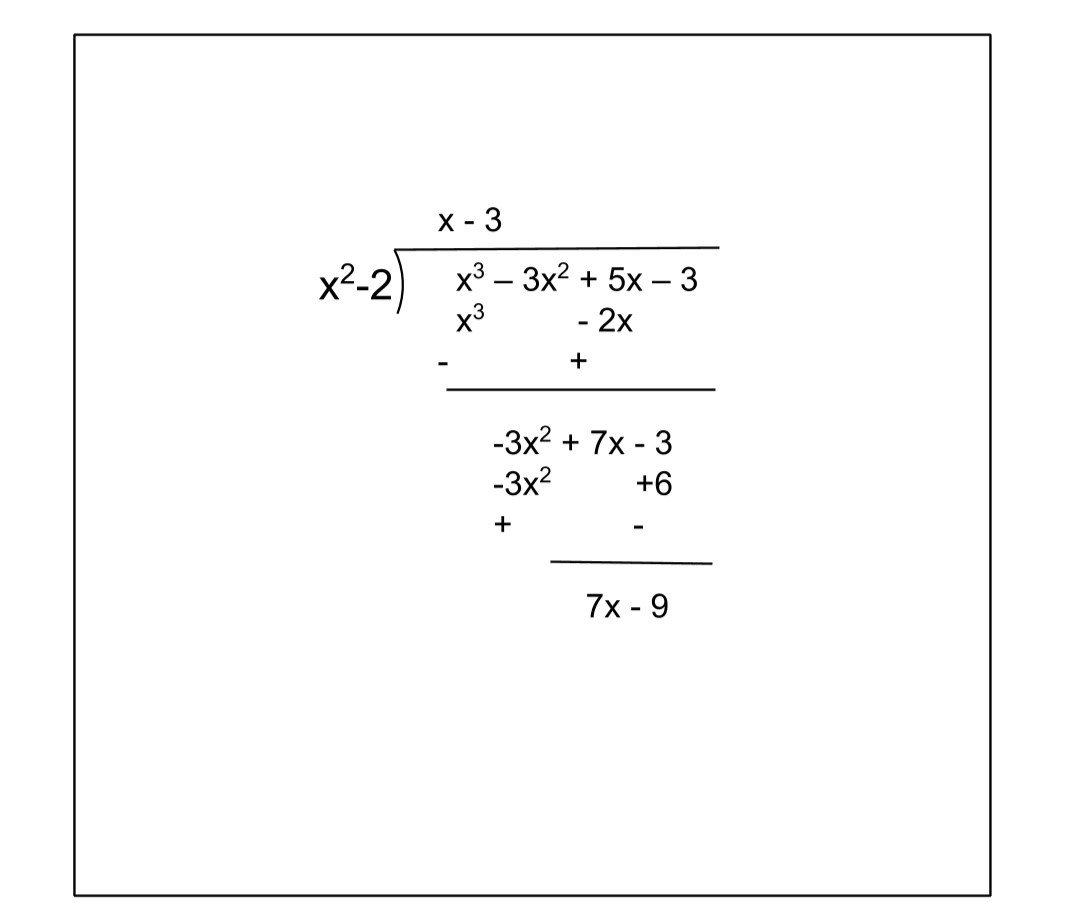Therefore, we get
Quotient = x – 3 and Remainder = 7x – 9.

(ii) p(x) = x4 – 3x2 + 4x + 5, g(x) = x2 + 1 – x = x2 – x +1
Applying long division as follows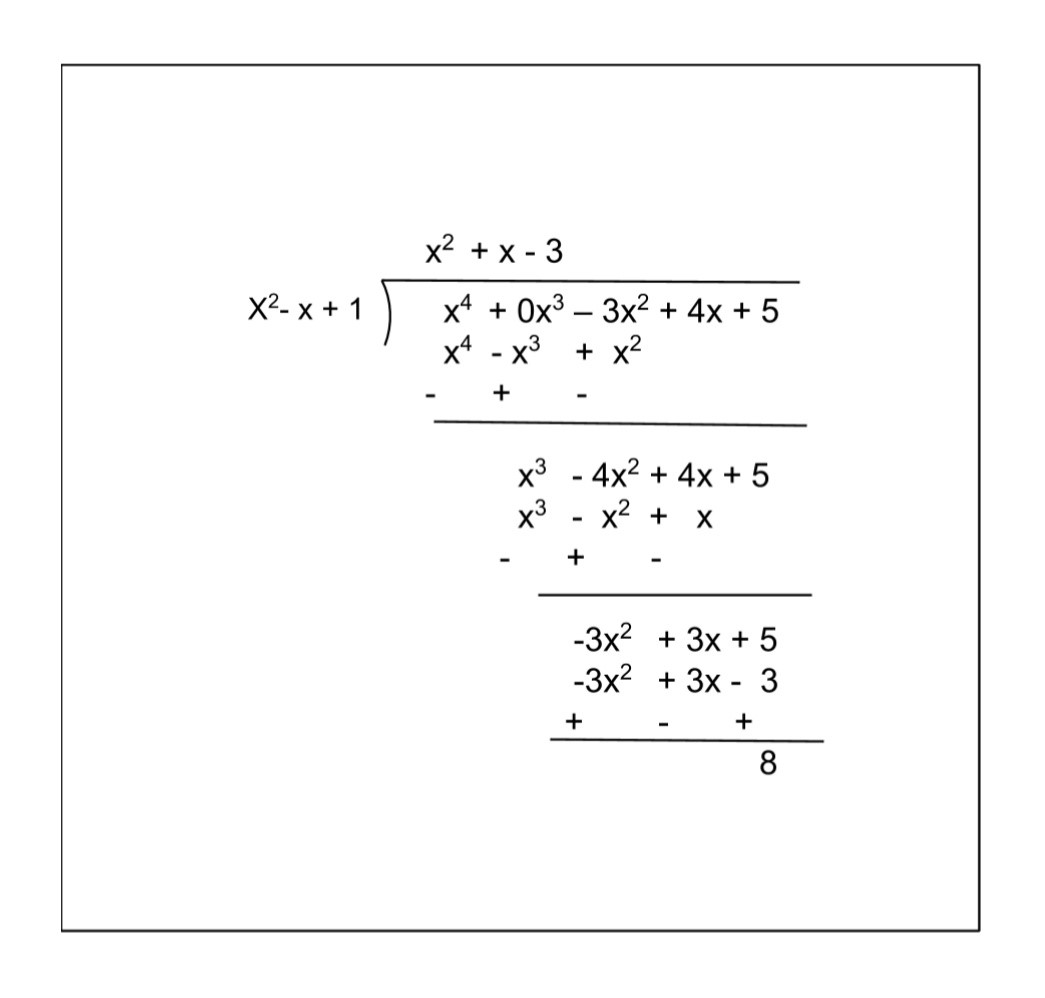Therefore, we get
Quotient = x2 + x – 3 and Remainder = 8.

(iii) p(x) = x4 – 5x + 6 = x4 + 0x2– 5x + 6
g(x) = 2 – x2 = – x2 + 2
Applying long division as follows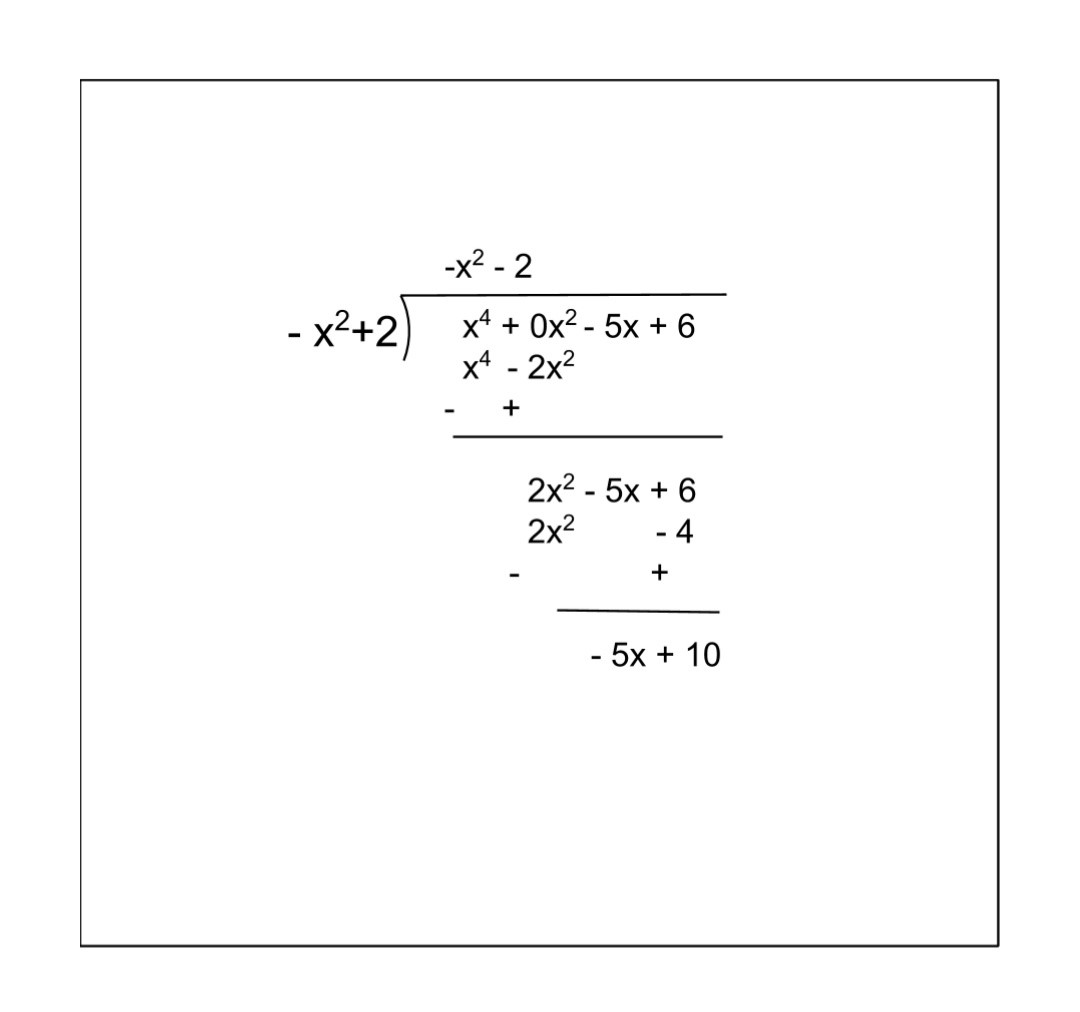Therefore, we get
Quotient = -x2 – 2 and Remainder = -5x + 10.

2. Check whether the first polynomial is a factor of the second polynomial by dividing the second polynomial by the first polynomial:
(i) t2 – 3, 2t4 + 3t3 – 2t2 – 9t – 12
(ii) x2 + 3x + 1, 3x4 + 5x3 – 7x2 + 2x + 2
(iii) x3 – 3x + 1, x5 – 4x3 + x2 + 3x + 1

Solution :

(i) Applying long division as follows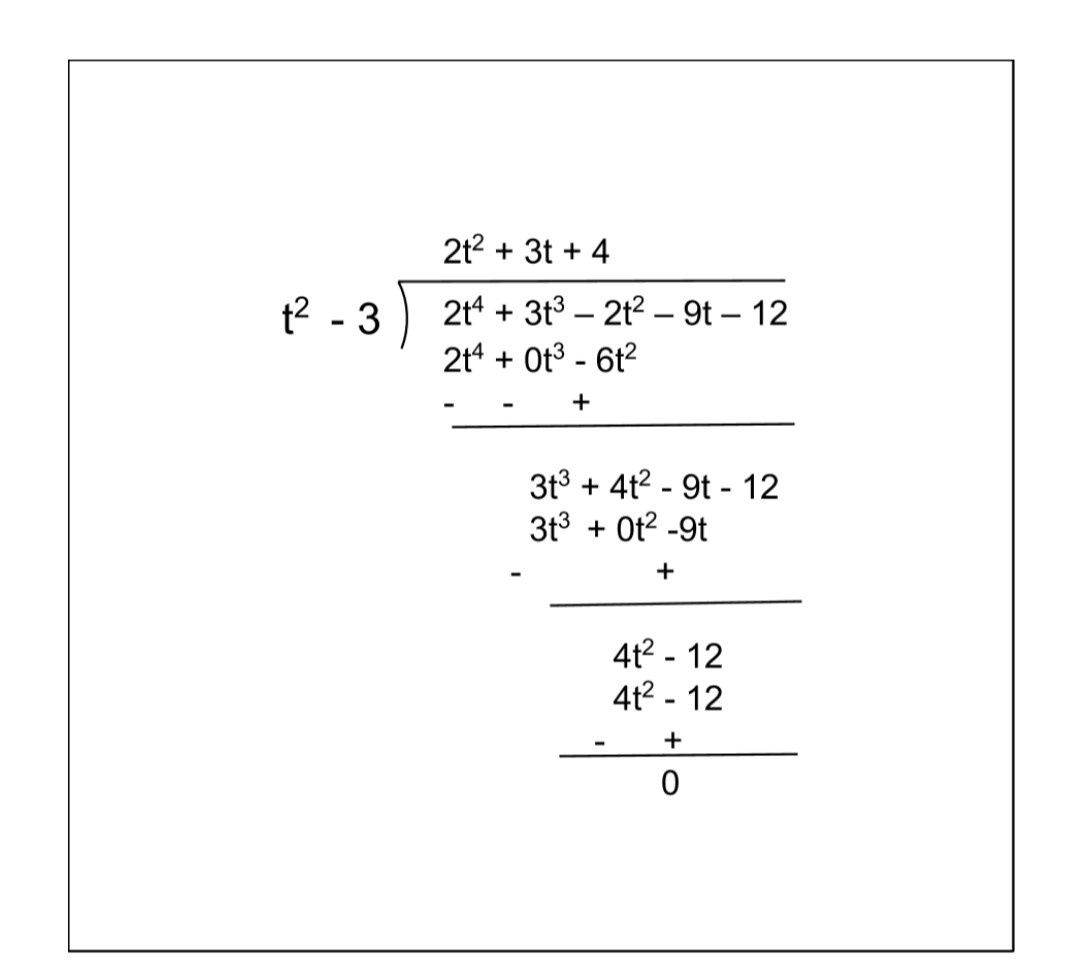Since we get, Remainder = 0, therefore the polynomial t2 – 3 is a factor of 2t4 + 3t3 – 2t2 – 9t – 12.

(ii) Applying long division as follows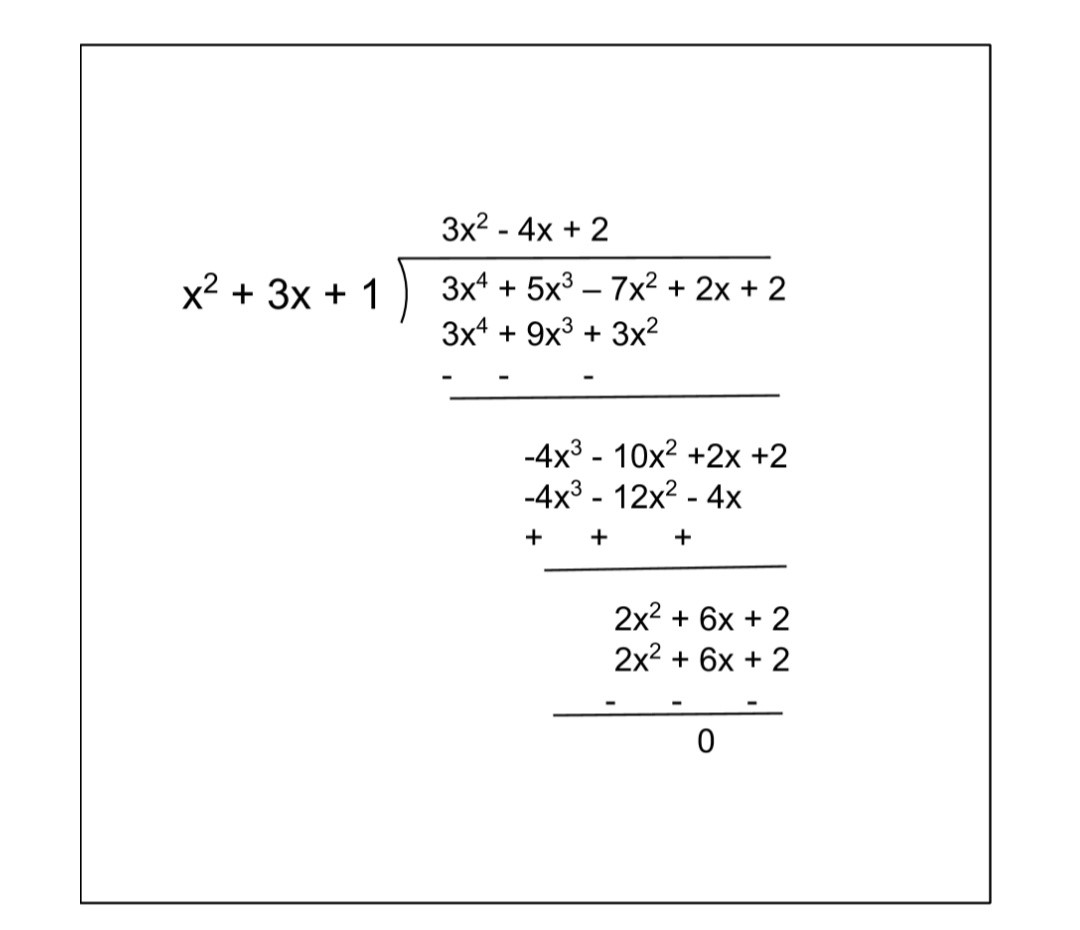Since we get, Remainder = 0, therefore the polynomial x2 + 3x + 1 is a factor of 3x4 + 5x3 – 7x2 + 2x + 2.

(iii) Applying long division as follows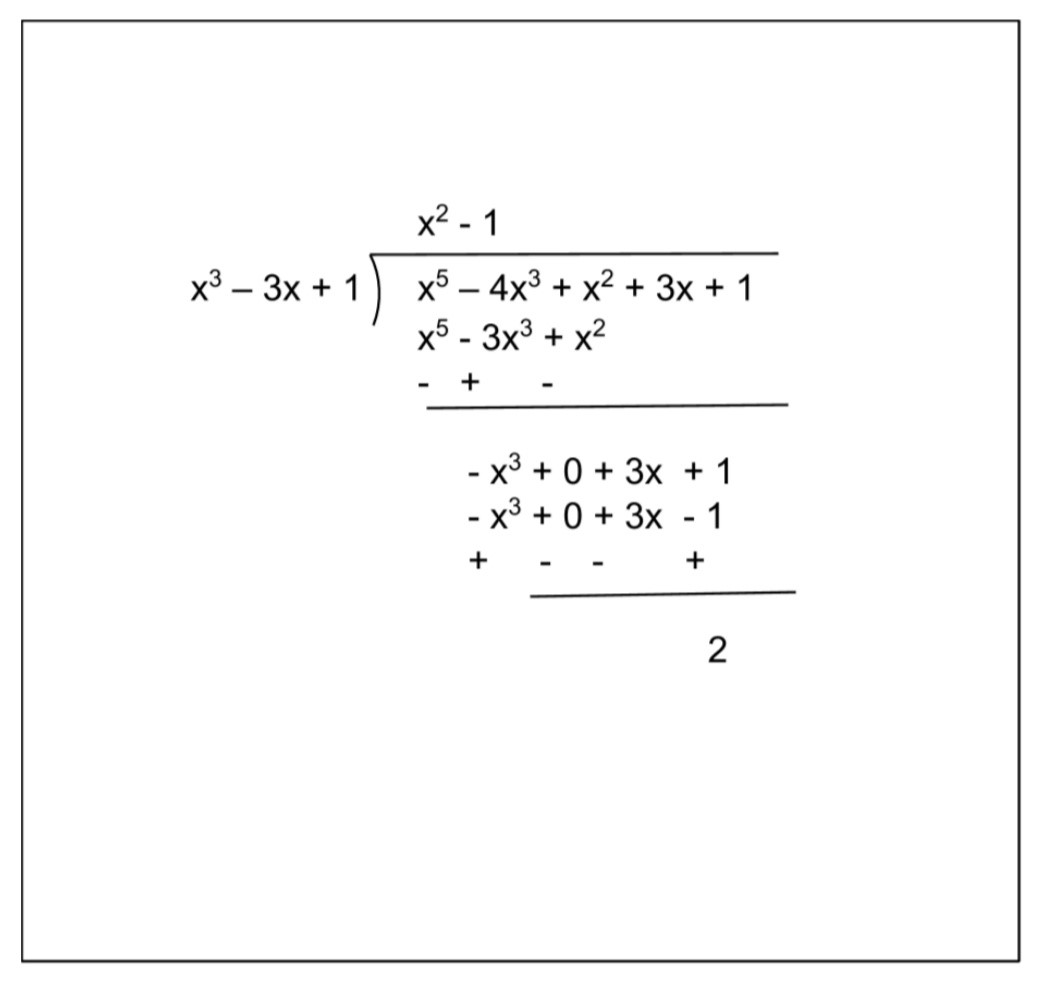Since we get, Remainder = 2, therefore the polynomial x3 – 3x + 1 is not a factor of x5 – 4x3 + x2 + 3x + 1.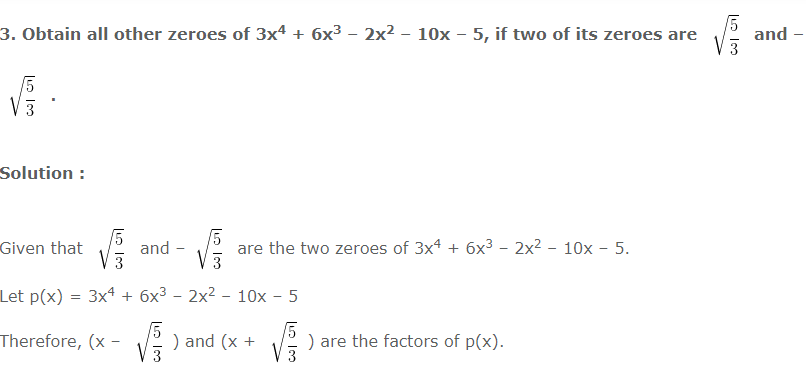Hence, (x2 – (5/3)) is a factor of p(x).
For the other two factors applying long division as follows :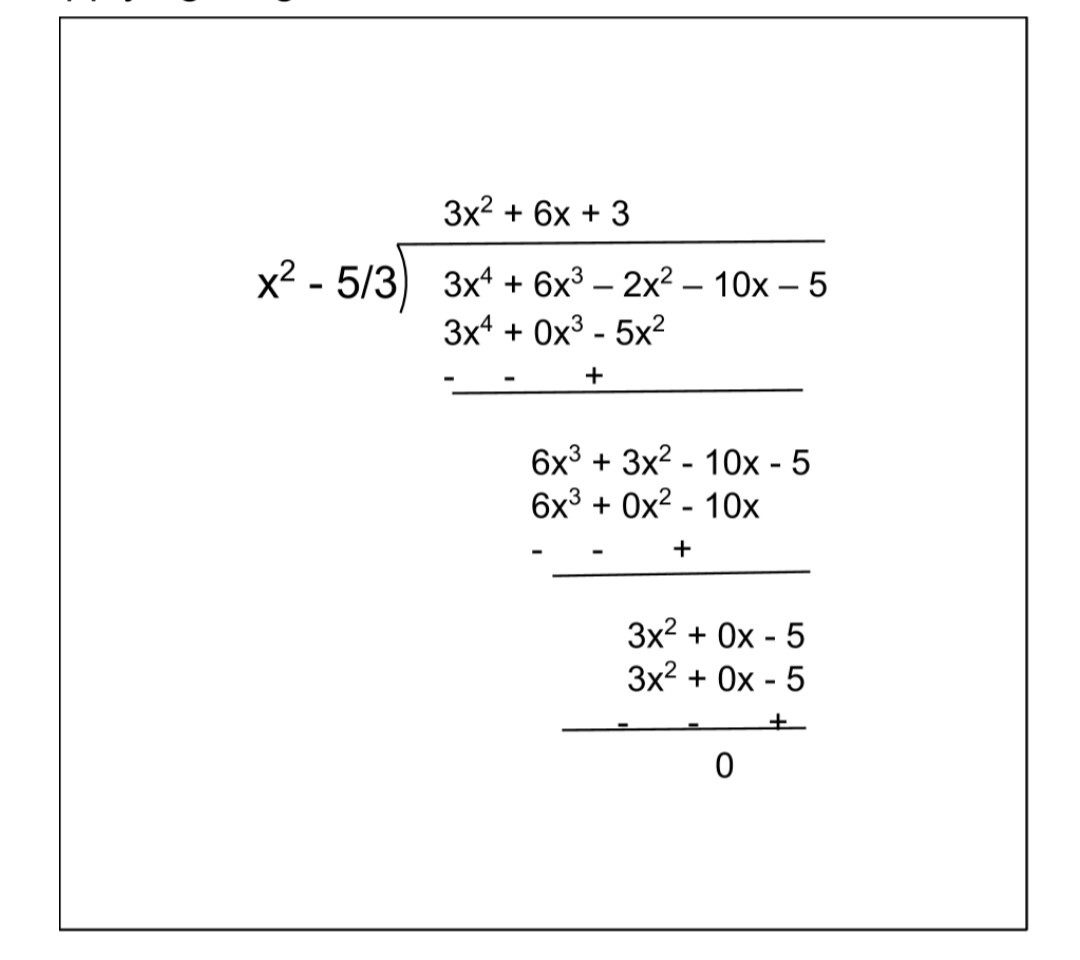Thus,
3x4 + 6x3 – 2x2 – 10x – 5
=  (x2 – (5/3)) (3x2 + 6x + 3)
= 3(x2 – (5/3)) (x2 + 2x + 1)
= 3(x2 – (5/3)) (x2 + x + x + 1)
= 3(x2 – (5/3)) (x(x+1) + 1(x + 1))
= 3(x2 – (5/3)) (x + 1)(x + 1)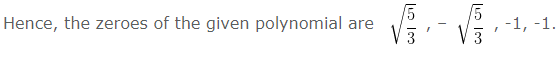4. On dividing x3 – 3x2 + x + 2 by a polynomial g(x), the quotient and remainder were x – 2 and –2x + 4, respectively. Find g(x).

Solution :

Given
Divisor = g(x)
Quotient = x – 2
Dividend = x3 – 3x2 + x + 2
Remainder = -2x + 4
We know that,
Dividend = Divisor x Quotient + Remainder
Therefore,
x3 – 3x2 + x + 2 = g(x).(x – 2) + (-2x + 4)
g(x).(x – 2) = (x3 – 3x2 + x + 2) – (-2x + 4)
g(x).(x – 2) = (x3 – 3x2 + 3x – 2)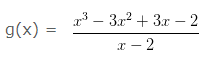Applying long division as follows :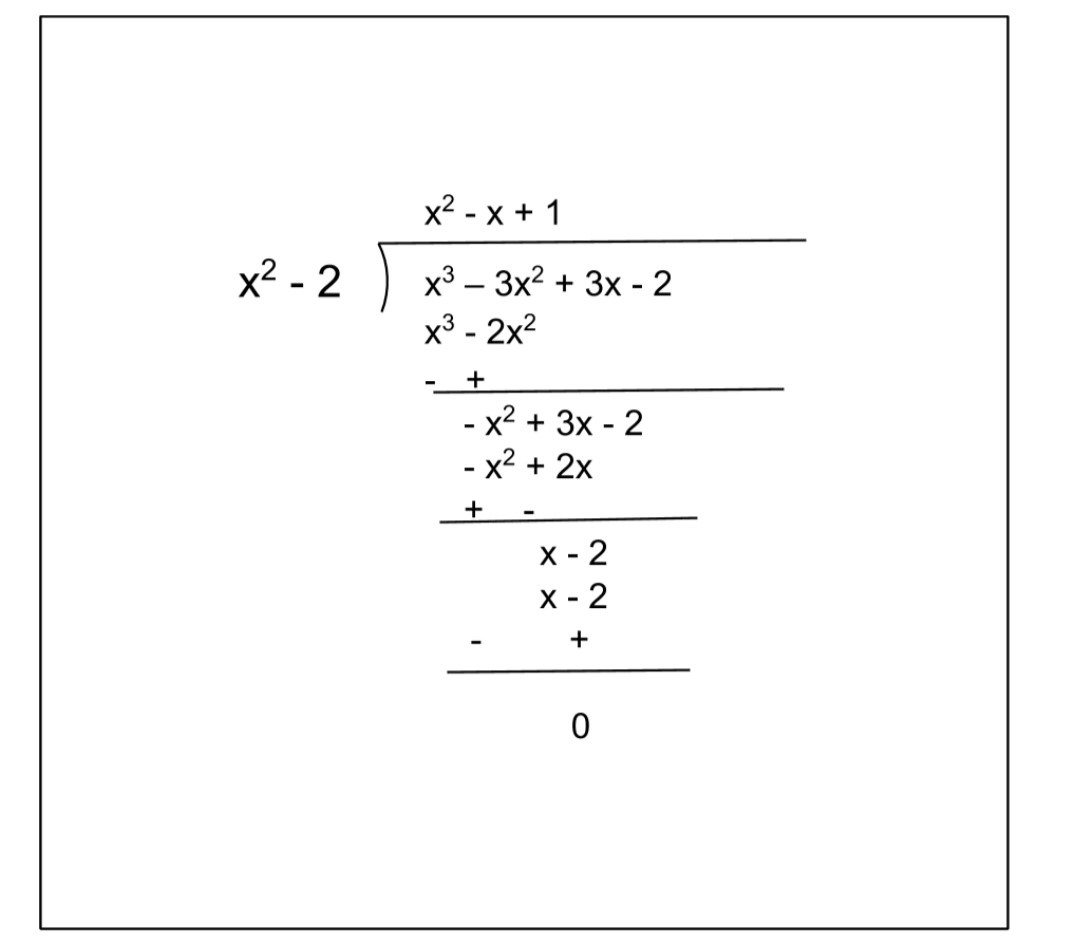g(x) = x2 – x + 1

5. Give examples of polynomials p(x), g(x), q(x) and r(x), which satisfy the division algorithm and

(i) deg p(x) = deg q(x)
(ii) deg q(x) = deg r(x)
(iii) deg r(x) = 0

Solution :

According to the division algorithm p(x) = g(x)q(x) + r(x) , where q(x) , degree of r(x) = 0 or degree of r(x) < degree of g(x).

(i) deg p(x) = deg q(x)
p(x) = 2x2 + 4x + 3
q(x) = x2 + 2x + 1
g(x) = 2
r(x) = 1
the given condition is satisfied.

(ii) deg q(x) = deg r(x)
p(x) = x4 + x
q(x) = x
g(x) = x3
r(x) = x
the given condition is satisfied.

(iii) deg r(x) = 0
p(x) = 2x2 + 4x + 3
q(x) = x2 + 2x + 1
g(x) = 2
r(x) = 1
the given condition is satisfied.

NCERT Solutions for Class 10 Maths Chapter 2 Exercise 2.3 – Polynomials, has been designed by the NCERT to test the knowledge of the student on the topic – Division Algorithm for Polynomials